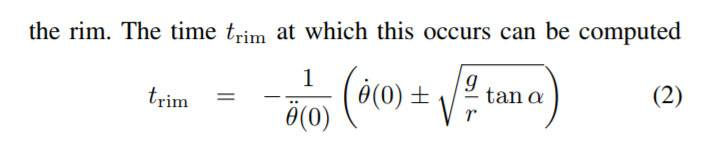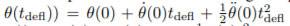# Roulette Prediction Algorithm Problem

• I
• shlomi123
If you're using the rim of the wheel as your reference, then ##\theta(0)## would be the angle of the ball from the center of the rim.You are not specifying what coordinate system you are using, so I can't help you with that.f

#### shlomi123

The roulette prediction algorithm is described here: http://pingless.co.il/roulette.pdf

And I have a problem with the formula attachedWhere teta θ is position, velocity and acceleration of the ball. g is garvity force. r is the ball radius from center of the roulette. a is stator incline.
According to my calculations:
TrimCoefficient = -1/-34.13 Inch/Sec^2
ballVelocity = 120 Inch/Sec
Gravity = 386.09 Inch/Sec^2
Rrim = 14 Inches
statorIncline = (28/180)*3.14;
Trim = TrimCoefficient*(ballVelocity+math.sqrt( (Gravity/Rrim)*math.tan(statorIncline) ));
I get Trim=3.6 and it should be as I think (if you count from the start if the ball spin) Trim=18 at least.

#### Attachments

And also if someone can help me to solve why the units don't fit
If you substitute all the units you get -S^2/I*(I/S + 1/S) = S+S/I
And it should be seconds only why you get +S/I

Angles should all be in radians.
##\dot\theta## should be in radians per second, but you're using a value in inches per second. And similarly for ##\ddot\theta##, it should be in radians/s^2 but you're using inches per second squared.

Since I suspect you are using linear velocity and linear acceleration at the rim, I think you need to divide by the radius to get the corresponding angular velocity and acceleration.

•berkeman
Hey thank you for your note,
this is my calculations after the radians / sec change:
Rrim = 14
TrimCoefficient = -1/(-30/Rrim)
print("TrimCoeff: "+str(TrimCoefficient))
ballVelocity = 88/Rrim
print("ballVelocity: "+str(ballVelocity))
# (65 - 88) / (1.36 - 1)
Gravity = 386.09
statorIncline = (28/180)*3.14;
Trim = TrimCoefficient*(ballVelocity+math.sqrt( (Gravity/Rrim)*math.tan(statorIncline) ));
print("Trim Trials: "+str(Trim))

and the result are:
TrimCoeff: 0.4666666666666667
ballVelocity: 6.285714285714286
Trim Trials: 4.719797943795565

Your original velocity and acceleration were 120 and -34.13, respectively. In your new calculation you are using 88 and -30. I assume you really did intend to work the calculation with different parameters the second time?

That formula seems to be the correct implementation of the one you quoted. Are you happy with the results?

Hey thank you, but I'm not happy from the results, Trim should be 18 as you can count from this movie
Because Trim is the time the ball is falling from the rim it completes about 11 circles and it takes 18 seconds and the formula says it takes only 4.7 seconds
What else could be wrong here

Hey I think the acceleration is wrong because -30 inch/sec should stop the ball at speed 90 inch/ sec in 3 seconds do you agree? So the actual acceleration should be -5-10 inch / sec

https://www.physicsforums.com/attachments/xxcc-jpg.232282/
This equation for the ball position of the roulette game taken from here: http://pingless.co.il/images/APCCAS08.pdf#page=3

This is my result:
Rrim = 14
ballPosition = (30/180)*3.14
ballVelocity = 88/Rrim (Convert inch/sec to rad/sec)
ballAcceleration = -8/Rrim (Convert inch/sec to rad/sec)
Tdefl = 18
ballAngle = float(ballPosition) + (ballVelocity*Tdefl) + 0.5*(ballAcceleration*Tdefl*Tdefl)

Last edited:
My guess is that the 21 radians means that the ball has orbited the wheel 3.34225 times. If its starting point was 0 degrees N then it would be ~123 degrees to the left of 0 degrees when it stopped.

Hey but actually it's not make sense the ball orbited the wheel only 3.34 times it should orbit 11 times and more as you can see from this movie:

Threads merged and re-opened. @shlomi123 -- Please do not start multiple threads on the same subject. Thank you.

Hey I still have a problem with this formulaWhich is from here: http://pingless.co.il/roulette.pdf#page=3

(1) I don't know from where in the wheel I should put the x-axis (zero angle) and (2) I don't know to define the θ(0) where the ball starts the orbit, please help me.

<< Mentor Note -- post edited to remove inappropriate content >>

#### Attachments

Last edited by a moderator:
As I read the initial graphic on that link, ##\theta## is the angle of the ball from the center of the wheel as reckoned in non-rotating coordinates. It does not matter what angle you take as ##\theta(0)## as long as you measure both starting and ending position using the same reference.